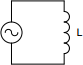# Inductive reactance calculator

## Calculates inductive reactance of an inductor

 Example 1: Must calculate the reactance of a 50uH inductor at 60khz View example
 Inductance henrys Frequency hertz Reactance ohmsYou may use one of the following SI prefix after a value: p=pico, n=nano, u=micro, m=milli, k=kilo, M=mega, G=giga

 Results Reactance ~18.849556 ohms